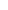Note: The other languages of the website are Google-translated. Back to Englishoror

## 根據 Excel 中的條件計算唯一數值#### 根據 Excel 2019、2016 及更早版本中的條件計算唯一數值

{=SUM(--(FREQUENCY(IF(criteria_range=criteria,range),range)>0))}
Array formula, should press Ctrl + Shift + Enter keys together.
• criteria_range：與您指定的條件匹配的單元格範圍；
• criteria：您要基於的唯一值計數的條件；
• range：要計數的具有唯一值的單元格的範圍。

=SUM(--(FREQUENCY(IF(A2:A12=E2,C2:C12),C2:C12)>0))##### 公式說明：

=SUM(--(FREQUENCY(IF(A2:A12=E2,C2:C12),C2:C12)>0))

• IF(A2:A12=E2,C2:C12)：如果 A 列中的產品是“T 卹”，則此 IF 函數返回 C 列中的值，結果是這樣的數組：{FALSE;300;500;FALSE;400;FALSE;300;FALSE;FALSE;錯誤；350}。
• FREQUENCY(IF(A2:A12=E2,C2:C12),C2:C12)= FREQUENCY({FALSE;300;500;FALSE;400;FALSE;300;FALSE;FALSE;FALSE;350},{200;300;500;350;400;450;300;550;200;260;350})：FREQUENCE函數用於對數組列表中的每個數值進行計數，並返回結果為：{0;2;1;1;1;0;0;0;0;0;0;0} .
• --(FREQUENCY(IF(A2:A12=E2,C2:C12),C2:C12)>0)=--({0;2;1;1;1;0;0;0;0;0;0;0}>0): 測試數組中的每個值是否都大於 0，並得到這樣的結果：{FALSE;TRUE;TRUE;TRUE;TRUE;FALSE;FALSE;FALSE;FALSE;FALSE;FALSE;FALSE}。 然後，雙負號將 TRUE 和 FALSE 轉換為 1 和 0，返回這樣的數組：{0;1;1;1;1;0;0;0;0;0;0;0}。
• SUM(--(FREQUENCY(IF(A2:A12=E2,C2:C12),C2:C12)>0))=SUM({0;1;1;1;1;0;0;0;0;0;0;0}): 最後，使用 SUM 函數將這些值相加，得到總數：4。

=SUM(--(FREQUENCY(IF((criteria,_range1=criteria1)* (criteria,_range2=criteria2)*…,range),range)>0))

#### 根據 Excel 365 中的條件計算唯一數值

=ROWS(UNIQUE(FILTER(range,criteria_range=criteria)))
• range：要計數的具有唯一值的單元格的範圍。
• criteria_range：與您指定的條件匹配的單元格範圍；
• criteria：您要基於的唯一值計數的條件；

=ROWS(UNIQUE(FILTER(C2:C12,A2:A12=E2)))##### 公式說明：

=ROWS(UNIQUE(FILTER(C2:C12,A2:A12=E2)))

• A2:A12=E2：此表達式檢查單元格 E2 中的值是否存在於 A2:A12 範圍內，並得到以下結果：{FALSE;TRUE;TRUE;FALSE;TRUE;FALSE;TRUE;FALSE;FALSE;FALSE;TRUE}。
• FILTER(C2:C12,A2:A12=E2)：FREQUENCE函數用於對數組列表中的每個數值進行計數，並返回結果為：{0;2;1;1;1;0;0;0;0;0;0;0} .
• UNIQUE(FILTER(C2:C12,A2:A12=E2))=UNIQUE({300;500;400;300;350})：這裡，UNIQUE 函數用於從列表數組中提取唯一值以獲得此結果：{300;500;400;350}。
• ROWS(UNIQUE(FILTER(C2:C12,A2:A12=E2)))=ROWS({300;500;400;350})：ROWS 函數返回基於單元格範圍或數組的行數，因此，結果為：4。

1. 如果數據范圍內不存在匹配的值，您將得到一個錯誤值，要將錯誤值替換為 0，請應用以下公式：

=IFERROR(ROWS(UNIQUE(FILTER(C2:C12,A2:A12=E2))), 0)2.要根據多個條件計算唯一值，您只需要將其他條件添加到帶有 * 字符的公式中，如下所示：

=ROWS(UNIQUE(FILTER(range,(criteria_range1=criteria1)* (criteria_range2=criteria2)*…)))

#### 使用的相對功能：

• 和：
• Excel SUM 函數返回提供的值的總和。
• 頻率：
• FREQUENCY 函數計算值在一個值範圍內出現的頻率，然後返回一個垂直的數字數組。
• 行：
• ROWS 函數返回給定引用或數組中的行數。
• 獨特的：
• UNIQUE 函數返回列表或範圍中的唯一值列表。
• 過濾：
• FILTER 功能有助於根據您定義的條件過濾一系列數據。

#### 更多文章：

• 計算列中的唯一數值或日期
• 假設您有一個包含一些重複項的數字列表，現在，您想要計算唯一值的數量，或者這些值在列表中只出現一次，如下面的屏幕截圖所示。 在本文中，我們將討論在 Excel 中快速輕鬆地解決此任務的一些有用公式。
• 計算兩列之間的所有匹配/重複項
• 比較兩列數據併計算兩列中的所有匹配項或重複項可能是我們大多數人的常見任務。 例如，您有兩列名稱，有些名稱同時出現在第一列和第二列中，現在，您要計算兩列之間所有匹配的名稱（位於兩列內任何位置的匹配項），如下圖所示，本教程將介紹一些在 Excel 中實現這一目標的公式。
• 計數單元格數等於多個值之一
• 假設，我在 A 列中有一個產品列表，現在，我想從 A 列中獲取列在 C4:C6 範圍內的特定產品 Apple、Grape 和 Lemon 的總數，如下圖所示。 通常，在 Excel 中，簡單的 COUNTIF 和 COUNTIFS 函數在這種情況下不起作用。 這篇文章，我將談談如何通過 SUMPRODUCT 和 COUNTIF 函數的組合快速輕鬆地解決這個工作。

### 最佳辦公效率工具

#### Kutools for Excel-幫助您從人群中脫穎而出

• 專為 1500 個工作場景而設計，幫助您解決 80% 的 Excel 問題。
• 每天減少數千次鍵盤和鼠標的點擊，減輕您疲倦的眼睛和手的疲勞。
• 在3分鐘內成為Excel專家。 不再需要記住任何痛苦的公式和VBA代碼。
• 30天無限制免費試用。 60天退款保證。 免費升級和支持2年。#### Office選項卡-在Microsoft Office（包括Excel）中啟用選項卡式閱讀和編輯

• 一秒鐘即可在數十個打開的文檔之間切換！
• 每天為您減少數百次鼠標單擊，告別鼠標手。
• 查看和編輯多個文檔時，將您的工作效率提高 50%。
• 像Chrome，Firefox和新的Internet Explorer一樣，為Office（包括Excel）帶來高效選項卡。Say something here...
symbols left.